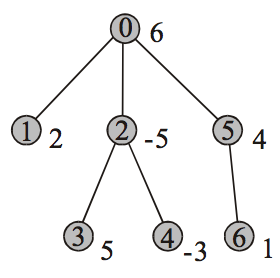시간 제한메모리 제한제출정답맞힌 사람정답 비율
5 초 128 MB329803618.090%

## 문제Consider a rooted tree, each of whose nodes is associated with an integer representing the node’s value. Naturally, the value of a subset of nodes of such a tree is regarded as the sum of the values of all the nodes contained in the subset. Given a positive integer K, let’s define the K-anti-parental subset to be a subset of nodes satisfying the following constraints: (1) the number of nodes in the subset is between 1 and K, inclusive, and (2) for any pair of nodes in the subset, one is not a parent of the other, and vice versa. Now, your task is to find the maximum of the values of the K-anti-parental subsets of an arbitrary node-valued tree.

## 입력

Your program is to read from standard input. The input consists of T test cases, where the positive integer T is given in the first line of the input, followed by the description of each test case. The first line of a test case contains two positive integers N and K, respectively indicating the number of nodes of the tree and the parameter K as explained above, in which we assume N ≤ 100,000 and 1 ≤ K ≤ 100. The tree’s nodes are indexed 0 to N-1, where the index 0 is always assigned to the root node. The following line contains N integers, separated by spaces, coming from the inclusive interval [-1,000, 1,000], which represent the values of the nodes enumerated in the increasing order of indices from 0 to N-1. The next following line contains N-1 integers, separated by spaces, each of which indicates the index of a corresponding node’s parent. Make sure that these numbers are for the N-1 nodes except the root, listed in the increasing order of indices from 1 (not 0) to N-1. Therefore, the first integer points to the parent of the node indexed 1. Note that the parent of the root node needs not be specified.

## 출력

Your program is to write to standard output. Print exactly one line per each test case. The line should contain the maximum possible value of the K-anti-parental subsets of the input tree.

## 예제 입력 1

3
7 3
6 2 -5 5 -3 4 1
0 0 2 2 0 5
3 2
4 -3 5
0 0
7 6
-1 -1 -1 -1 -1 -1 -1
0 1 2 3 4 5


## 예제 출력 1

12
5
-1